# Problem: An ideal monatomic gas is contained in a cylinder with a movable piston so that the gas can do work on the outside world, and heat can be added or removed as necessary. The figure shows various paths that the gas might take in expanding from an initial state whose pressure, volume, and temperature are , , and  respectively. The gas expands to a state with final volume . For some answers it will be convenient to generalize your results by using the variable , which is the ratio of final to initial volumes (equal to 4 for the expansions shown in the figure.)The figure shows several possible paths of the system in the pV plane. Although there are an infinite number of paths possible, several of those shown are special because one of their state variables remains constant during the expansion. These have the following names:Adiabiatic: No heat is added or removed during the expansion.Isobaric: The pressure remains constant during the expansion.Isothermal: The temperature remains constant during the expansion.Part CWhich of the curves in the figure represents an isothermal process?ABCD

###### FREE Expert Solution

Part C

Isothermal process condition is given as:

pV = K

P1V1 = P2V2

It should be a parabolic curve, which is either B or C or D.

For B:

P1V1 = (1.00)(1.0) = 1.00

95% (403 ratings)###### Problem Details

An ideal monatomic gas is contained in a cylinder with a movable piston so that the gas can do work on the outside world, and heat can be added or removed as necessary. The figure shows various paths that the gas might take in expanding from an initial state whose pressure, volume, and temperature are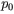,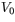, and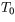respectively. The gas expands to a state with final volume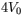. For some answers it will be convenient to generalize your results by using the variable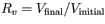, which is the ratio of final to initial volumes (equal to 4 for the expansions shown in the figure.)

The figure shows several possible paths of the system in the pV plane. Although there are an infinite number of paths possible, several of those shown are special because one of their state variables remains constant during the expansion. These have the following names:

• Isobaric: The pressure remains constant during the expansion.
• Isothermal: The temperature remains constant during the expansion.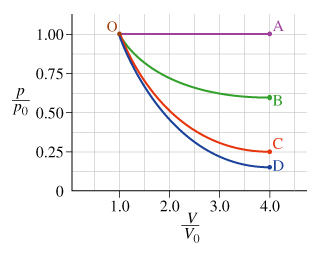Part C

Which of the curves in the figure represents an isothermal process?

A

B

C

D